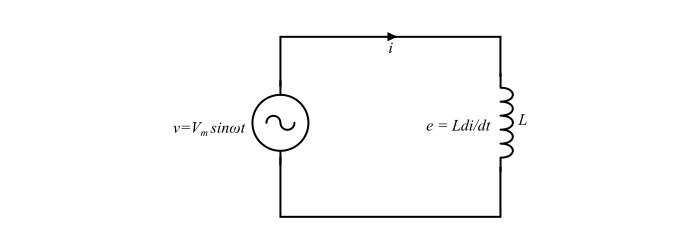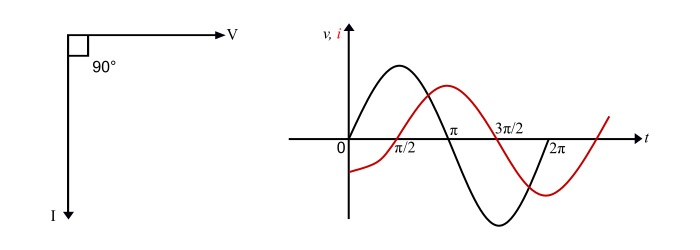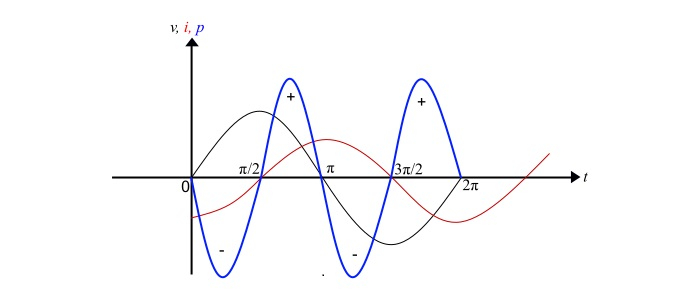# Inductors in AC Circuits

Electronics & ElectricalElectronDigital Electronics

Consider the circuit containing a pure inductive coil of inductance L Henry. When an alternating voltage V (RMS) is applied across the coil, an alternating current I (RMS) will flow through the circuit. Due to this alternating current, a back emf (e) is induced in the coil due to inductance of it. This back emf at every instant opposes the any change in current through the coil.Let the applied alternating voltage is

$$\mathrm{\nu=V_{m}sin\:\omega t}\:\:\:… (1)$$

The back emf (e) induced in the inductor coil is given by,

$$\mathrm{e=L \frac{di}{dt}}\:\:\:… (2)$$

Since, there is no ohmic drop, thus the applied voltage has to overcome the back emf only. Therefore,

Applied voltage = Back emf

$$\mathrm{V_{m}\:sin\:\omega t =L \frac{di}{dt}}$$

$$\mathrm{di=\frac{V_{m}}{L}sin\: \omega t dt}$$

Integrating on both sides, we obtain,

$$\mathrm{i=\frac{V_{m}}{L}\:\int \:sin\:\omega t\:dt=\frac{V_{m}}{L}(\frac{-cos\:\omega t}{\omega})=\frac{V_{m}}{\omega\:L}(-cos\:\omega t)}$$

$$\mathrm{\Rightarrow\:i=\frac{V_{m}}{\omega\:L}sin(\omega t-90^{\circ})}\:\:\:… (3)$$

In the equation (3), when sin (ωt - 90°) = 1, the value of i will be maximum. Therefore,

$$\mathrm{I_{m}=\frac{V_{m}}{\omega\:L}}\:\:\:… (4)$$

Thus, the equation (3) becomes,

$$\mathrm{i=I_{m}sin(\omega t-90^{\circ})}\:\:\:… (5)$$

From the equation (5), it is clear that, the current through pure inductor lags behind the voltage across the inductor by 90°. It can also be represented using phasor diagram and waveform as,## Inductive Reactance

Since, we have seen that

$$\mathrm{I_{m}=\frac{V_{m}}{\omega\:L}}$$

$$\mathrm{\Rightarrow\:\frac{V_{m}}{I_{m}}=\omega\:L=X_{L}}$$

$$\mathrm{\Rightarrow\:X_{L}=\omega\:L=2\pi\:fL=\frac{V_{m}/ \sqrt{2}}{I_{m}\sqrt{2}}=\frac{V}{I}}\:\:\:… (6)$$Where, V and I are the RMS values of voltage and current respectively. The ω = 2πfL is the angular supply frequency.

Hence, the XL is the opposition offered by inductor to the flow of electric current. It is known as inductive reactance of the inductive coil. The XL is measured in ohms(Ω).

## Power

• Instantaneous Power − The instantaneous power (p) is given by the product of instantaneous voltage and instantaneous current as follows,

$$\mathrm{p=\nu×i=V_{m}sin \:\omega\:t×I_{m}sin(\omega\:t-90^{\circ})=-V_{m}I_{m}sin\:\omega t\:cos\:\omega t}$$

$$\mathrm{\Rightarrow\:p=-\frac{V_{m}\:I_{m}}{2}sin\:2\omega t}\:\:\:… (7)$$

• Average Power − The average power (P) is the mean of instantaneous power over one cycle. Thus,

$$\mathrm{p=\frac{1}{2\pi}\int_{0}^{2\pi}-\frac{V_{m}\:I_{m}}{2}sin\:2\omega t\:d\omega t}\:\:\:… (8)$$Hence, the average power absorbed in the pure inductor is zero over one cycle. The power curve over one cycle shows that positive power is equal to negative power. So, the resultant power over one cycle is zero i.e. no power consumed by the inductor. The electrical power only flows from the source to coil and back again.

## Numerical Example

A current of 20 A flows through a pure inductive coil, when it is connected across a 250 V, 50 Hz supply. Determine the following −

• Inductive reactance
• Inductance of the coil
• Power absorbed
• Equations for voltage and current.

Solution

• Inductive reactance

$$\mathrm{X_{L}=\frac{V}{I}=\frac{250}{20}= 12.5 Ω}$$

• Inductance of the coil

$$\mathrm{∵X_{L}=2\pi fL}$$

$$\mathrm{\Rightarrow L=\frac{X_{L}}{2\pi f}=\frac{12.5}{2\pi×50}=0.0398 H = 39.8 mH}$$

• Power absorbed – Since, the given coil is a pure inductor. Therefore, power absorbed is zero.
• Equations for voltage and current

$$\mathrm{Maximum\:values\:of\:voltage,V_{m}=√2 × V=√2 × 250 = 353.5 V}$$

$$\mathrm{Maximum\:values\:of\:current,I_{m}=\frac{V_{m}}{X_{L}}=\frac{353.5}{12.5}= 28.28 A}$$

$$\mathrm{Angular frequency,\omega=2\pi f=2\pi× 50 = 314 rad⁄sec}$$

Therefore,

$$\mathrm{Equation\:of\:voltage,\nu= 353.5\:sin 314t}$$

$$\mathrm{Equation\:of\:current,t= 28.28\:sin(314t− 90^{\circ})}$$